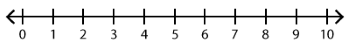##### Notes

Daily Math Practice provides a week's worth of problems ideal for morning work or review. Week 24 reviews standards from previous weeks and supports Common Core Mathematics Standards K.OA.A.1, K.OA.A.2, & K.OA.A.3.

##### Print Instructions

NOTE: Only your test content will print.
To preview this test, click on the File menu and select Print Preview.

See our guide on How To Change Browser Print Settings to customize headers and footers before printing.

# Kindergarten - Daily Math Practice - Week 24 (Kindergarten)

Print Test (Only the test content will print)
 DAILY MATH PRACTICE - WEEK 24 DAY 1 DAY 2 Add. Use the number line.3 + 5 = Subtract. Use the number line.10 - 3 = Mrs. Gray has 9 pencils. She gives away 4 pencils. How many pencils does Mrs. Gray have left?Cross off the correct number of pictures:Answer:pencils There are 6 children playing tag. Then, 2 more children join them. How many children are playing tag now?Write an addition sentence:Answer:children Circle the addition sentence for the model.4 + 1 = 53 + 2 = 5 Circle the addition sentence for the model.5 + 2 = 76 + 1 = 7 Circle the smaller number8 or 3 Draw a B above the A. Draw a C next to the A.A

 DAILY MATH PRACTICE - WEEK 24 DAY 3 DAY 4 Add the dots.+5 + 4 = Subtract the dots.-6 - 2 = A baker makes 8 muffins. She sells 7 of the muffins. How many muffins are left?Cross off the correct number of pictures:Answer:muffins There are 5 red apples in a basket. There are also 5 green apples in the basket. How many apples in the basket?Write an addition sentence:Answer:apples Circle the addition sentence for the model.6 + 3 = 93 + 6 = 9 Circle the addition sentence for the model.1 + 5 = 62 + 4 = 6 Start at 37. Count forward to 43. Circle the number that comes next.37, 38, 39, 40, 41, 42, 43, 44, 45, 46 Color the two shapes with the same number of sides.You need to be a HelpTeaching.com member to access free printables.